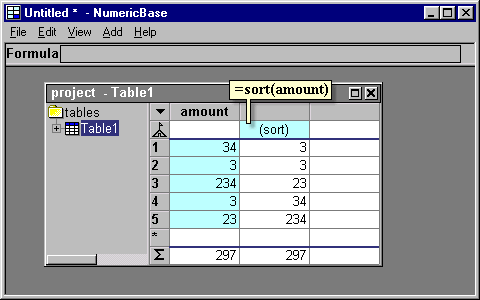# summary functions

Build in summary functions.
The build-in summary functions let you perform a summary of all the data rows of a table. Each function gets a specific number of arguments, evaluates these arguments separately for each row, and performs some specific calculation on the results.

The following table shows some of the summary functions. Each summary function expects values of certain type (shows in "expected type" column). If a value is not of this type, it is converted to it automatically.

 summary function Expected type Meaning concat(x) Text value Concatenation sum(x) Numeric value Numerical summary _or(x) Logical value "or" logical operation _and(x) Logical value "and" logical operation min(x) Numeric value Numerical minimum max(x) Numeric value Numerical maximum avg(x) Numeric value Numerical average wavg(value,weight) Numeric value Weighted average
Table: summary functions

Using summary functions locally.
You can use the summary functions to summarize a set of values locally. To do that, you need to select one of the summary function that has only one parameter (for example, wavg is not qualified). Then, you give it more than one parameter.

When you doe this, the functions does not scans all the data rows of the table. Instead, it scans the arguments and perform the required operation. For example, =sum(3,4,5) evaluates to 12 because 3+4+5 equals to 12.

summary functions with more than one output value
Some summary functions produces more than one output value. More specifically, they have one output value for each row of the table. You can use them at the base row - the values are shown in the same column across the different rows of the table.

For example, the sort summary function can be used to produce a column that have all the values of another column in sorted order - see the following screenshot for an example. Note that it works independently from the sorting functionality introduced in the "Overview" sectionScreenshot: the "sort" summary function

Other function like this is mavg(values, num_points) , which let you produce a moving average.